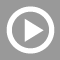#Videos

## Microsoft Excel VideosExcel's built-in statistical functions can be supplemented with the Analysis Toolpak. If you are not sure how to load an Add-In into Excel, this video demonstrates the simple technique and shows you how to get started with the Analysis Toolpak....How to do a multiple regression analysis of data in an Excel worksheet. If you have not done so already, you will need to add-in the analysis toolpak as described in that video. This video is basic "Yellow-belt" Excel.Excel's Scenario Manager conveniently keeps track of several different sets of values that can be plugged into a worksheet to examine the consequence of changes. For example, we might want to examine growth under several potential changes in unemployment...In this video, I use fake data from Lesson 1 to do summary statistics in Excel. Using a fake continuous variable, we calculate mean (average), standard deviation, count of observations, and then calculate a standard error. From this, we develop a...In this video, I show tips for making fake data. Fake data are useful for testing your understanding of certain statistical concepts, but are not actually that easy to make. The fake data made in this video will be used in subsequent videos to demonstrate...